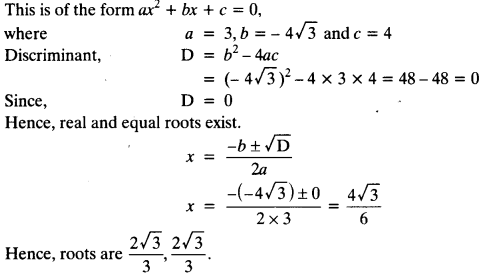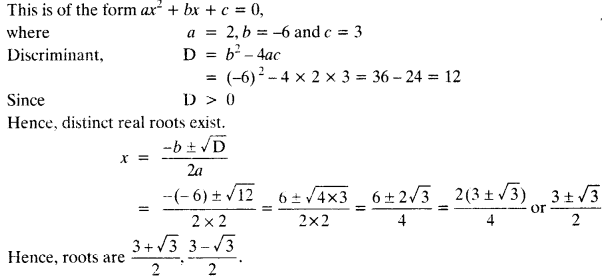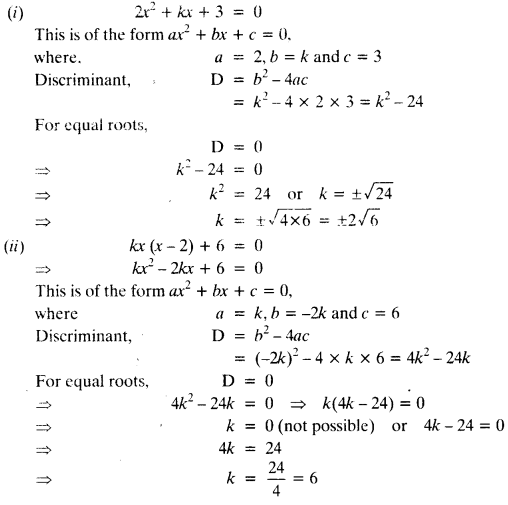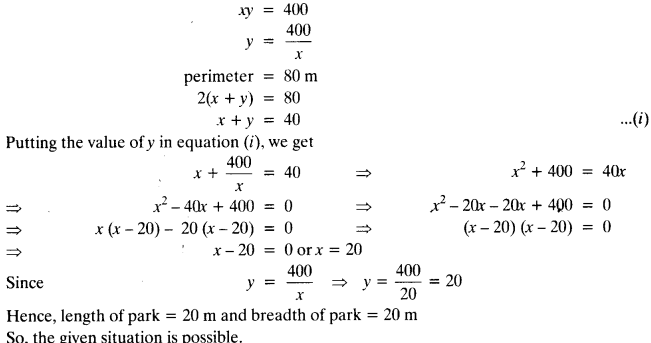# NCERT Solutions for Class 10 Maths Chapter 4 Quadratic Equations Ex 4.4

NCERT Solutions for Class 10 Maths Chapter 4 Quadratic Equations Ex 4.4 are part of NCERT Solutions for Class 10 Maths. Here we have given NCERT Solutions for Class 10 Maths Chapter 4 Quadratic Equations Ex 4.4. https://mcqquestions.guru/ncert-solutions-for-class-10-maths-chapter-4-ex-4-4/

 Board CBSE Textbook NCERT Class Class 10 Subject Maths Chapter Chapter 4 Chapter Name Quadratic Equations Exercise Ex 4.4 Number of Questions Solved 5 Category NCERT Solutions

## NCERT Solutions for Class 10 Maths Chapter 4 Quadratic Equations Ex 4.4

Ex 4.4 Class 10 Maths Question 1.
Find the nature of the roots of the following quadratic equations. If the real roots exist, find them:
(i) 2x² -3x + 5 = 0
(ii) 3x2 – 4√3x + 4 = 0
(iii) 2x2-6x + 3 = 0
Solution:
(i) 2x2 – 3x + 5 = 0
This is of the form ax2 + bx + c = 0,
where a = 2, b = -3 and c = 5
Discriminant, D = b2-4ac
= (-3) 2-4 x 2 x 5 = 9 – 40 = -31
Since, D < 0
Hence, no real roots exist.

(ii) 3x2 – 4√3x + 4 = 0(iii) 2x2-6x + 3 = 04.4 Class 10 Maths Question 2.
Find the values of k for each of the following quadratic equations, so that they have two equal roots.
(1) 2x2 + kx + 3 = 0
(2) kx (x – 2) + 6 = 0
Solution:Exercise 4.4 Class 10 Maths Question 3.
Is it possible to design a rectangular mango grove whose length is twice its breadth, and the area is 800 m2? If so, find its length and breadth.
Solution:
Let breadth of the rectangular mango grove be x m
Then, the length of rectangular mango grove be 2xm
According to question,
x x 2x = 800
⇒ 2x2 = 800
⇒ x2 = 400
⇒ x = ±20 [-20 is rejected]
Hence, breadth = 20 m and length = 2 x 20 = 40 m
So, it is possible to design a rectangular mango grove whose length is twice its breadth.

Ex4.4 Class 10 Maths Question 4.
Is the following situation possible? If so, determine their present ages.
The sum of the ages of two friends is 20 years. Four years ago, the product of their ages in years was 48.
Solution:
Let the present age of one friend be x years
Then, the present age of other friend be (20 -x) years
4 years ago, one friend’s age was (x – 4) years
4 years ago, other friend’s age was (20 -x – 4) = (16 -x) years
According to question,
(x-4) (16-x) = 48
⇒ 16x – x2 – 64 + 4x = 48
⇒ x2 – 20x + 112 = 0
This is of the form ax2 + bx + c = 0, where, a = 1, b = -20 and c = 112
Discriminant, D = b2 – 4ac
= (-20)2 – 4 x 1 x 112 = 400 – 448 = – 48 < 0
Since, no real roots exist.
So, the given situation is not possible.

Class 10 Maths Chapter 4 Exercise 4.4 Question 5.
Is it possible to design a rectangular park of perimeter 80 m and area 400 m2? If so, find its length and breadth.
Solution:
Let the length of rectangular park be x m and breadth be y m.
Given: area = 400 m2We hope the NCERT Solutions for Class 10 Maths Chapter 4 Quadratic Equations Ex 4.4 help you. If you have any query regarding NCERT Solutions for Class 10 Maths Chapter 4 Quadratic Equations Ex 4.4, drop a comment below and we will get back to you at the earliest.